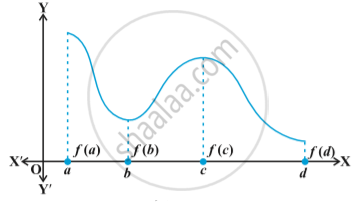# Maximum and Minimum Values of a Function in a Closed Interval

#### notes

Let us consider a function  f  given by
f(x) = x + 2, x ∈ (0, 1)
The function is continuous on (0, 1) and neither has a maximum value nor has a minimum value. Further, we may note that the function even has neither a local maximum value nor a local minimum value.

However, if we extend the domain of  f  to the closed interval [0, 1], then f still may not have a local maximum (minimum) values but it certainly does have maximum value 3 = f(1) and minimum value 2 =  f(0). The maximum value 3 of f at x = 1 is called absolute maximum value (global maximum or greatest value) of f on the interval [0, 1]. Similarly, the minimum value 2 of f  at x  = 0 is called the absolute minimum value (global minimum or least value) of f on [0, 1].

Consider the graph given in  following Fig .  a continuous function defined on a closed interval [a, d]. Observe that the function f has a local minima at x = b and localminimum value is f(b). The function also has a local maxima at x = c and local maximum value is f (c).

Also from the graph, it is evident that f has  absolute maximum value f(a) and absolute minimum value f(d). Further note that the absolute maximum (minimum) value of f is different from local maximum (minimum) value of f.

#### theorem

Let f  be a continuous function on an interval I = [a, b]. Then f has the absolute maximum value and f attains it at least once in I. Also, f has the absolute minimum value and attains it at least once in I.

#### theorem

Let f be a differentiable function on a closed interval I and let c be any interior point of I. Then

(i) f′(c) = 0 if  f attains its absolute maximum value at c.

(ii) f′(c) = 0 if  f attains its absolute minimum value at c.
In view of the above results, we have the following working rule for finding absolute maximum and/or absolute minimum values of a function in a given closed interval [a, b].

Working Rule -

Step 1: Find all critical points of  f  in the interval, i.e., find points x where either ( ) 0f x =′ or f  is not differentiable.

Step 2: Take the end points of the interval.

Step 3: At all these points (listed in Step 1 and 2), calculate the values
of  f .

Step 4: Identify the maximum and minimum values of  f  out of the values calculated in Step 3. This maximum value will be the absolute maximum (greatest) value of f  and the minimum value will be the absolute minimum (least) value of  f .

If you would like to contribute notes or other learning material, please submit them using the button below.

### Shaalaa.com

Maxima Minima Part 3 (Local Maxima and Minima) [00:48:12]
S
0%